## When doing blood testing for a viral infection, the procedure can be made more efficient and less expensive by combining partial samples of

Question

When doing blood testing for a viral infection, the procedure can be made more efficient and less expensive by combining partial samples of different blood specimens. If samples from three people are combined and the mixture tests negative, we know that all three individual samples are negative. Find the probability of a positive result for three samples combined into one mixture, assuming the probability of an individual blood sample testing positive for the virus is 0.06.

in progress 0
3 months 2021-09-05T08:49:25+00:00 1 Answers 0 views 0

0.1694 = 16.94% probability of a positive result for three samples combined into one mixture.

Step-by-step explanation:

For each test, there are only two possible outcomes. Either it is positive, or it is negative. The probability of a test being positive or negative is independent of any other test, which means that the binomial probability distribution is used to solve this question.

Binomial probability distribution

The binomial probability is the probability of exactly x successes on n repeated trials, and X can only have two outcomes.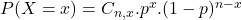In which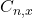is the number of different combinations of x objects from a set of n elements, given by the following formula.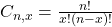And p is the probability of X happening.

The probability of an individual blood sample testing positive for the virus is 0.06.

This means that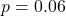If samples from three people are combined and the mixture tests negative, we know that all three individual samples are negative. Find the probability of a positive result for three samples combined into one mixture.

It will be positive if at least one of the tests is positive, that is: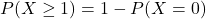In which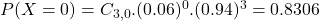Then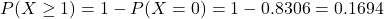0.1694 = 16.94% probability of a positive result for three samples combined into one mixture.